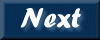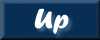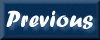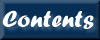Next: 9. Common Parameters Up: XRONOS User's Guide Previous: 7. Output File Format   Contents

Subsections

# 8. QDP/PLT

The array of results from each XRONOS task can be plotted with QDP/PLT, an all purpose plotting and function fitting program. It operates on ASCII files containing both the data and plotting command used to control the display. The plotting function is available for the following tasks : autocor, crosscor, efold, efsearch, lcurve, powspec and timeskew.

# 8.1 Overview

QDP reads the ASCII file containing various plotting commands and the data, then calls the PLT subroutines which then executes the commands and plot the data. At this point the PLT> prompt appears and the user can then proceed to perform the following:

• Display information about the interactive commands via HElp.
• Override various PLT defaults.
• Override the default PLT commands
• Plot data with various combinations of lines, markers, and error bars.
• Change the appearance/style of the of the plot, for example converting all text into Roman Font.
• Plot the data as a function of a different x variable.
• Change the number of panels in which the data is plotted.
• Define models and to calculate the 'best fit' parameter values.
• Generate hardcopy.

QDP/PLT can be run interactively within each XRONOS application by entering commands at the PLT> prompt, or can automatically execute commands from a script with the hidden parameter plotfile.

# 8.2 Useful QDP Commands

A few, commonly used QDP/PLT commands are given below; the full descriptions of the PLT commands are in the "QDP/PLT User's Guide" or can be listed on the terminal by typing ? or help from the PLT prompt.

Rescaling: to rescale X and Y axes use:

```    PLT> r x xlow xhigh
PLT> r y ylow yhigh
PLT> r xlow xhigh ylow yhigh
```
Logarithmic Axes: to make the x and/or y axes logarithmic (to the base 10) use:

```    PLT> log x
PLT> log y
```
To turn off the logarithmic axes use

```    PLT> log off
```
Device: to change the current plot device use:

```    PLT> dev /xxx
```
where /xxx is the new plot device (e.g. /xw,/gif,/ps).

Modelling and Fitting: to use the fitting capability of QDP/PLT two are the main commands MOdel and FIT. The MOdel command is used to define the model, any combination of model components can be added together. For example:

```    PLT> mo cons linr quad
```
will add a constant, a linear and a quadratic term. The list of the available models is given typing:

```    PLT> mo ?
```
The Fit command cause PLT to call the fitting routine to search for the best fitting parameters for the model specified with the MOdel command.

Replot: after making changes in the PLT environment, a replot will show the effects of the modifications

```    PLT> p
```

Hardcopy: a hardcopy of the current plot is obtained using:

```    PLT> hardcopy filename
```
The file is in postscript.

EXit: to exit from the PLT subroutine type:

```    PLT> exit
```
QDP was written and is currently maintained by Allyn Tennant, at the Marshall Space Flight Center. PGPLOT, provided and maintained by Tim Pearson, is the graphics package used by QDP/PLT.

# 8.3 Plotting Multiple Series

For programs that handle simultaneously more than one time series (e.g. lcurve and efold) different types of analysis result can be plotted depending on the number of input time series. For two input time series, three different plots are provided (1) the sum of the intensities of the two input series on the X-axis and on the Y-axis the count ratio (ser2/ser1) of the two input series (Hardness-plot); (2) Time (or Phase) on the X-axis and the intensity on the Y-axis for the two input time series; (3) As (2) but also the ratio is plotted. For three or four input time series two different plots are available: (1) Colour-Colour diagram that shows the ratio of ser3/ser2 or ser4/ser3 on the Y-axis (for 3 or 4 input series respectively) and ser2/ser1 (always) on the X-axis; (2) Time (or Phase) on the X-axis and the intensity on the Y-axis for the 3 (or 4) input time series.

The choice of the plot type is done by setting the parameter plotdnum. Note that in the efold plot with Phase on the X-axis, the Y-axis can be either count/s or count/s normalised by average source intensity. Note that for the multicolor tasks all the arrays calculated by lcurve or efold are available in PLT>. A color ratio plot versus time or phase can be obtained as follows. At the prompt:

```     Two plotting styles available:
Colour-Colour  ; Intensity vs Time (or Phase)[ # of series (3 or 4)];
Enter PLOT style number (default=1)[]
```
Enter the number of time series. On the screen the plot has as many panels as many input series, showing the counts for each series on the Y-axis and time or phase on the X-axis.At the PLT> prompt type info which gives the list of arrays available. For example for 3 input series the info command gives :

```    PLT> info
Scales:
Grp  Wind    Label     XData Min    XData Max    YData Min    YData Max
1    -1  Time (s)   :  3496.    ,   8646.     :  3496.    ,   8646.
2     2  Ser 1      :  3496.    ,   8646.     :  331.8    ,   466.4
3     3  Ser 2      :  3496.    ,   8646.     :  415.9    ,   613.8
4     4  Ser 3      :  3496.    ,   8646.     :  747.7    ,   1080.
5    -1  Ser 2/Ser  :  3496.    ,   8646.     :  1.222    ,   1.325
6    -1  Ser 3/Ser  :  3496.    ,   8646.     :  1.755    ,   1.819
7    -1  Ser 1+2+3  :  3496.    ,   8646.     :  1495.    ,   2160.
8    -1             :  3496.    ,   8646.     :  1.000    ,   1.000
PLT>
```
To plot the ratios (array number 5 and 6) versus time together with the three input lightcurves use the following commands:

```    PLT> color 1 on 5 6
PLT> plot ver
PLT> plot
```
The final plot will contain 5 panels. The first three are the input series the last two the ratios.Next: 9. Common Parameters Up: XRONOS User's Guide Previous: 7. Output File Format   Contents